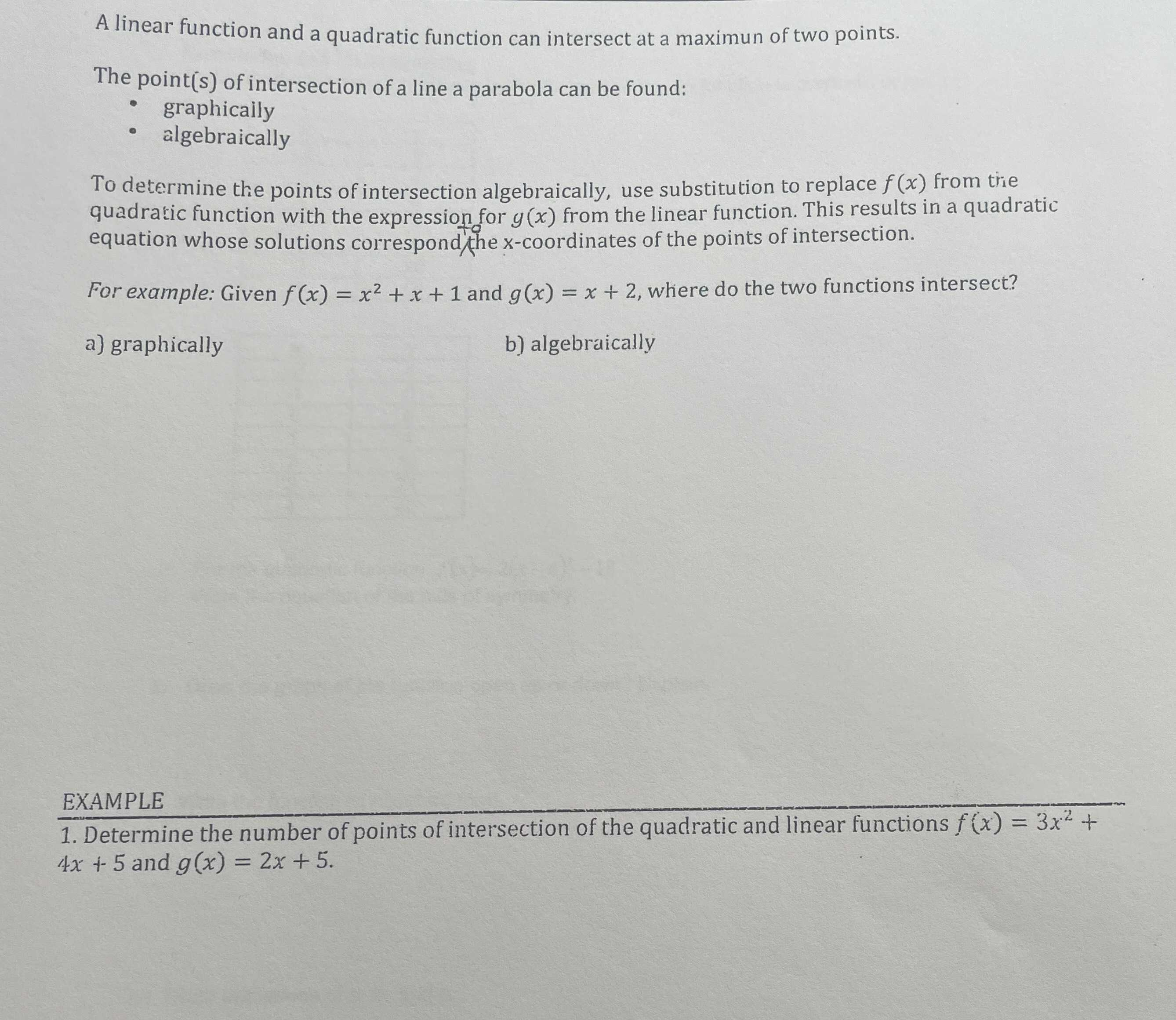### ¿Todavía tienes preguntas de matemáticas?

Pregunte a nuestros tutores expertos
Algebra
PreguntaA linear function and a quadratic function can intersect at a maximun of two points.

The point(s) of intersection of a line a parabola can be found:

graphicaily

algebraically

To determine the points of intersection algebraically, use substitution to replace $$f ( x )$$ from trie quadratic function with the expression for $$y ( x )$$ from the linear function. This results in a quadratic equation whose solutions correspondthe x-coordinates of the points of intersection.

For example: Given $$f ( x ) = x ^ { 2 } + x + 1$$ and $$g ( x ) = x + 2$$ , where do the two functions intersect?

a) graphically         b) algebraically